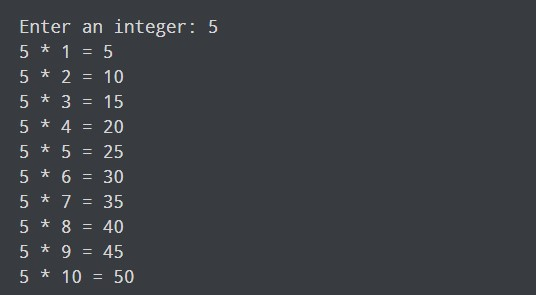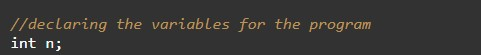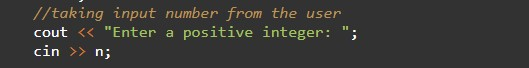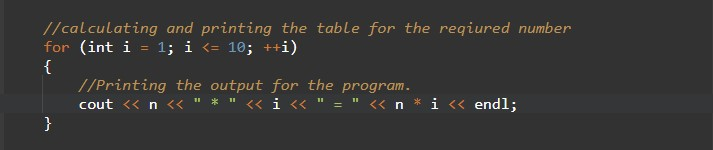# C++ Program to Generate Multiplication Table

In this tutorial you will learn about the C++ Program to Generate Multiplication Table and its application with practical example.

## C++ Program to Generate Multiplication Table

In this tutorial, we will learn to create a C++ program that will Generate a Multiplication Table in C++ programming.

## Prerequisites

Before starting with this tutorial we assume that you are best aware of the following C++ programming topics:

• Operators in C++ Programming.
• Basic Input and Output function in C++ Programming.
• Basic C++ Programming.

## Programfor generating the table:-

As we all know the integer, float, or double value in c++ programming. In c++ programming, we can take the input from the user in numbers format. In today’s program, we will take input in number format. Secondly, we will generate a Multiplication Table with the help of a small program. The numbers are the most useful terminology in any programing language. For number/arithmetic manipulation in c++ programming, there are many predefined functions available.

## Output:-In the above program, we have first initialized the required variable.• n = it will hold the input number value from the user.
• i  = it will hold the integer value.

Taking Input numbers from the user.Calculating the table of given numbers and Printing the output.In this tutorial we have learn about the C++ Program to Generate Multiplication Table and its application with practical example. I hope you will like this tutorial.